# Open Addressing | Linear Probing | Collision

## Collision Resolution Techniques-

Before you go through this article, make sure that you have gone through the previous article on Collision Resolution Techniques.

We have discussed-

• Hashing is a well-known searching technique.
• Collision occurs when hash value of the new key maps to an occupied bucket of the hash table.
• Collision resolution techniques are classified as-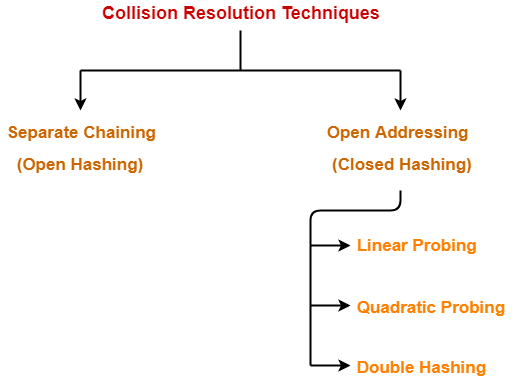• Unlike separate chaining, all the keys are stored inside the hash table.
• No key is stored outside the hash table.

Techniques used for open addressing are-

• Linear Probing
• Double Hashing

## Operations in Open Addressing-

Let us discuss how operations are performed in open addressing-

### Insert Operation-

• Hash function is used to compute the hash value for a key to be inserted.
• Hash value is then used as an index to store the key in the hash table.

In case of collision,

• Probing is performed until an empty bucket is found.
• Once an empty bucket is found, the key is inserted.
• Probing is performed in accordance with the technique used for open addressing.

### Search Operation-

To search any particular key,

• Its hash value is obtained using the hash function used.
• Using the hash value, that bucket of the hash table is checked.
• If the required key is found, the key is searched.
• Otherwise, the subsequent buckets are checked until the required key or an empty bucket is found.
• The empty bucket indicates that the key is not present in the hash table.

### Delete Operation-

• The key is first searched and then deleted.
• After deleting the key, that particular bucket is marked as “deleted”.

## NOTE-

• During insertion, the buckets marked as “deleted” are treated like any other empty bucket.
• During searching, the search is not terminated on encountering the bucket marked as “deleted”.
• The search terminates only after the required key or an empty bucket is found.

## Open Addressing Techniques-

Techniques used for open addressing are-

## 1. Linear Probing-

In linear probing,

• When collision occurs, we linearly probe for the next bucket.
• We keep probing until an empty bucket is found.

• It is easy to compute.

• The main problem with linear probing is clustering.
• Many consecutive elements form groups.
• Then, it takes time to search an element or to find an empty bucket.

### Time Complexity-

 Worst time to search an element in linear probing is O (table size).

This is because-

• Even if there is only one element present and all other elements are deleted.
• Then, “deleted” markers present in the hash table makes search the entire table.

## 2. Quadratic Probing-

• When collision occurs, we probe for i2‘th bucket in ith iteration.
• We keep probing until an empty bucket is found.

## 3. Double Hashing-

In double hashing,

• We use another hash function hash2(x) and look for i * hash2(x) bucket in ith iteration.
• It requires more computation time as two hash functions need to be computed.

## Comparison of Open Addressing Techniques-

Yes No No
Yes Yes No
m m m2
##### Cache performance
Best Lies between the two Poor

### Conclusions-

• Linear Probing has the best cache performance but suffers from clustering.
• Quadratic probing lies between the two in terms of cache performance and clustering.
• Double caching has poor cache performance but no clustering.

## Load Factor (α)-

Load factor (α) is defined as-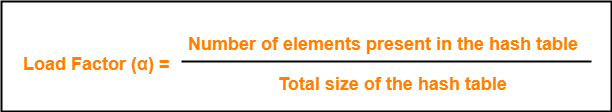In open addressing, the value of load factor always lie between 0 and 1.

This is because-

• In open addressing, all the keys are stored inside the hash table.
• So, size of the table is always greater or at least equal to the number of keys stored in the table.

## Problem-

Using the hash function ‘key mod 7’, insert the following sequence of keys in the hash table-

50, 700, 76, 85, 92, 73 and 101

Use linear probing technique for collision resolution.

## Solution-

The given sequence of keys will be inserted in the hash table as-

### Step-01:

• Draw an empty hash table.
• For the given hash function, the possible range of hash values is [0, 6].
• So, draw an empty hash table consisting of 7 buckets as-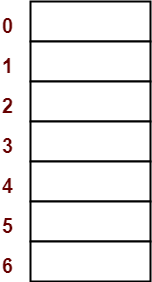### Step-02:

• Insert the given keys in the hash table one by one.
• The first key to be inserted in the hash table = 50.
• Bucket of the hash table to which key 50 maps = 50 mod 7 = 1.
• So, key 50 will be inserted in bucket-1 of the hash table as-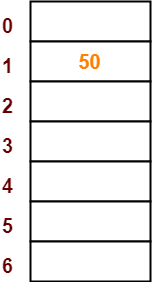### Step-03:

• The next key to be inserted in the hash table = 700.
• Bucket of the hash table to which key 700 maps = 700 mod 7 = 0.
• So, key 700 will be inserted in bucket-0 of the hash table as-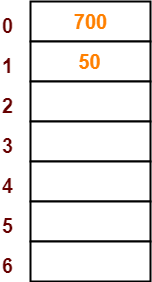### Step-04:

• The next key to be inserted in the hash table = 76.
• Bucket of the hash table to which key 76 maps = 76 mod 7 = 6.
• So, key 76 will be inserted in bucket-6 of the hash table as-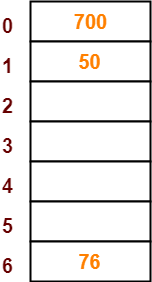### Step-05:

• The next key to be inserted in the hash table = 85.
• Bucket of the hash table to which key 85 maps = 85 mod 7 = 1.
• Since bucket-1 is already occupied, so collision occurs.
• To handle the collision, linear probing technique keeps probing linearly until an empty bucket is found.
• The first empty bucket is bucket-2.
• So, key 85 will be inserted in bucket-2 of the hash table as-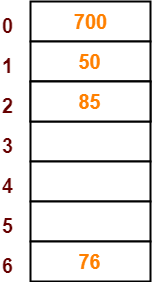### Step-06:

• The next key to be inserted in the hash table = 92.
• Bucket of the hash table to which key 92 maps = 92 mod 7 = 1.
• Since bucket-1 is already occupied, so collision occurs.
• To handle the collision, linear probing technique keeps probing linearly until an empty bucket is found.
• The first empty bucket is bucket-3.
• So, key 92 will be inserted in bucket-3 of the hash table as-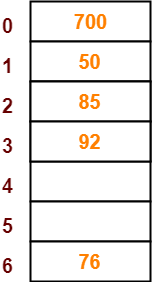### Step-07:

• The next key to be inserted in the hash table = 73.
• Bucket of the hash table to which key 73 maps = 73 mod 7 = 3.
• Since bucket-3 is already occupied, so collision occurs.
• To handle the collision, linear probing technique keeps probing linearly until an empty bucket is found.
• The first empty bucket is bucket-4.
• So, key 73 will be inserted in bucket-4 of the hash table as-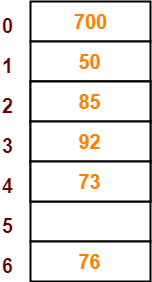### Step-08:

• The next key to be inserted in the hash table = 101.
• Bucket of the hash table to which key 101 maps = 101 mod 7 = 3.
• Since bucket-3 is already occupied, so collision occurs.
• To handle the collision, linear probing technique keeps probing linearly until an empty bucket is found.
• The first empty bucket is bucket-5.
• So, key 101 will be inserted in bucket-5 of the hash table as-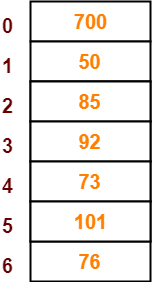To gain better understanding about Open Addressing,

Watch this Video Lecture

Next Article- Separate Chaining Vs Open Addressing

Get more notes and other study material of Data Structures.

Watch video lectures by visiting our YouTube channel LearnVidFun.

SummaryArticle Name
Open Addressing | Linear Probing | Collision
Description
Open Addressing is a collision resolution technique used for handling collisions in hashing. Techniques Used- Linear Probing, Quadratic Probing, Double Hashing. In linear probing, the next bucket is linearly probed.
Author
Publisher Name
Gate Vidyalay
Publisher Logo